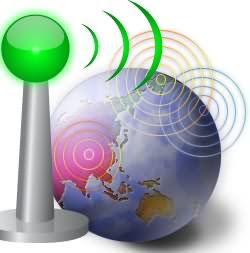## Wednesday, June 3, 2009

### What is the Meaning of Word Bandwidth?

The word Bandwidth has several related meanings, here is the list of means of Bandwidth in several phases.• Bandwidth (Computing) or digital bandwidth
A rate of data transfer, throughput or bit rate, measured in bits per second

• Bandwidth (signal processing) or analog bandwidth, frequency bandwidth or radio bandwidth:
A measure of the width of a range of frequencies, measured in hertz

• Spectral line bandwidth:
The width of an atomic or molecular spectral line, measured in hertz

Bandwidth can also refer to some other specific areas too:

• Bandwidth (linear algebra)
The width of the band of nonzero terms around the diagonal of a matrix

• In kernel density estimation
Bandwidth describes the width of the convolution kernel used